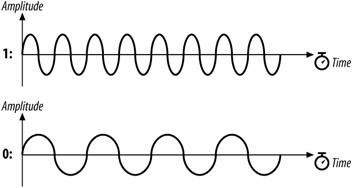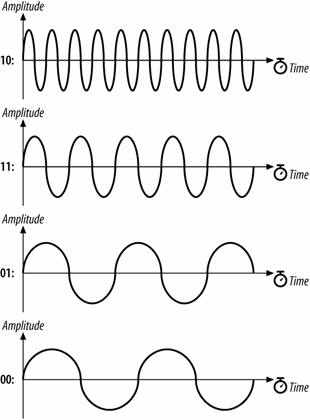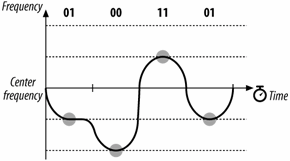###ผู้เขียน หัวข้อ: Gaussian Frequency Shift Keying (GFSK)  (อ่าน 5519 ครั้ง)

0 สมาชิก และ 1 บุคคลทั่วไป กำลังดูหัวข้อนี้

#### HS5TQA

• สมาชิกรุ่นกลาง
•• กระทู้: 106##### Gaussian Frequency Shift Keying (GFSK)
« เมื่อ: เมษายน 19, 2015, 12:59:43 PM »
Gaussian Frequency Shift Keying (GFSK)

The FH PHY uses Gaussian frequency shift keying (GFSK).
• Frequency shift keying encodes data as a series of frequency changes in a carrier. One advantage of using frequency to encode data is that noise usually changes the amplitude of a signal; modulation systems that ignore amplitude (broadcast FM radio, for example) tend to be relatively immune to noise. The Gaussian in GFSK refers to the shape of radio pulses; GFSK confines emissions to a relatively narrow spectral band and is thus appropriate for secondary uses. Signal processing techniques that prevent widespread leakage of RF energy are a good thing, particularly for secondary users of a frequency band. By reducing the potential for interference, GFSK makes it more likely that 802.11 wireless LANs can be built in an area where another user has priority.
• The term keying is a vestige of telegraphy. Transmission of data across telegraph lines required the use of a key. Sending data through a modern digital system employs modulation techniques instead, but the word keying persists.
2-Level GFSK

The most basic GFSK implementation is called 2-level GFSK (2GFSK). Two different frequencies are used, depending on whether the data that will be transmitted is a 1 or a 0. To transmit a 1, the carrier frequency is increased by a certain deviation. Zero is encoded by decreasing the frequency by the same deviation. Figure 11-5 illustrates the general procedure. In real-world systems, the frequency deviations from the carrier are much smaller; the figure is deliberately exaggerated to show how the encoding works.

The rate at which data is sent through the system is scalled the symbol rate. Because it takes several cycles to determine the frequency of the underlying carrier and whether 1 or 0 was transmitted, the symbol rate is a very small fraction of the carrier frequency. Although the carrier frequency is roughly 2.4 billion cycles per second, the symbol rate is only 1 or 2 million symbols per second.Figure 11-5. 2-level GFSK

Frequency changes with GFSK are not sharp changes. Instantaneous frequency changes require more expensive electronic components and higher power. Gradual frequency changes allow lower-cost equipment with lower RF leakage. Figure 11-6 shows how frequency varies as a result of encoding the letter M (1001101 binary) using 2GFSK. Note that the vertical axis is the frequency of the transmission. When a 1 is transmitted, the frequency rises to the center frequency plus an offset, and when a 0 is transmitted, the frequency drops by the same offset. The horizontal axis, which represents time, is divided into symbol periods. Around the middle of each period, the receiver measures the frequency of the transmission and translates that frequency into a symbol. (In 802.11 frequency-hopping systems, the higher-level data is scrambled before transmission, so the bit sequence transmitted to the peer station is not the same as the bit sequence over the air. The figure illustrates how the principles of 2GFSK work and doesn't step through an actual encoding.)Figure 11-6. GFSK encoding of the letter M

4-Level GFSK

Using a scheme such as this, there are two ways to send more data: use a higher symbol rate or encode more bits of information into each symbol. 4-level GFSK (4GFSK) uses the same basic approach as 2GFSK but with four symbols instead of two. The four symbols (00, 01, 10, and 11) each correspond to a discrete frequency, and therefore 4GFSK transmits twice as much data at the same symbol rate. Obviously, this increase comes at a cost: 4GFSK requires more complex transmitters and receivers. Mapping of the four symbols onto bits is shown in Figure 11-7.Figure 11-7. Mapping of symbols to frequencies in 4GFSK

With its more sophisticated signal processing, 4GFSK packs multiple bits into a single symbol. Figure 11-8 shows how the letter M might be encoded. Once again, the vertical axis is frequency, and the horizontal axis is divided into symbol times. The frequency changes to transmit the symbols; the frequencies for each symbol are shown by the dashed lines. The figure also hints at the problem with extending GFSK-based methods to higher bit rates. Distinguishing between two levels is fairly easy. Four is harder. Each doubling of the bit rate requires that twice as many levels be present, and the RF components distinguish between ever-smaller frequency changes. These limitations practically limit the FH PHY to 2 Mbps.Figure 11-8. 4GFSK encoding of the letter M